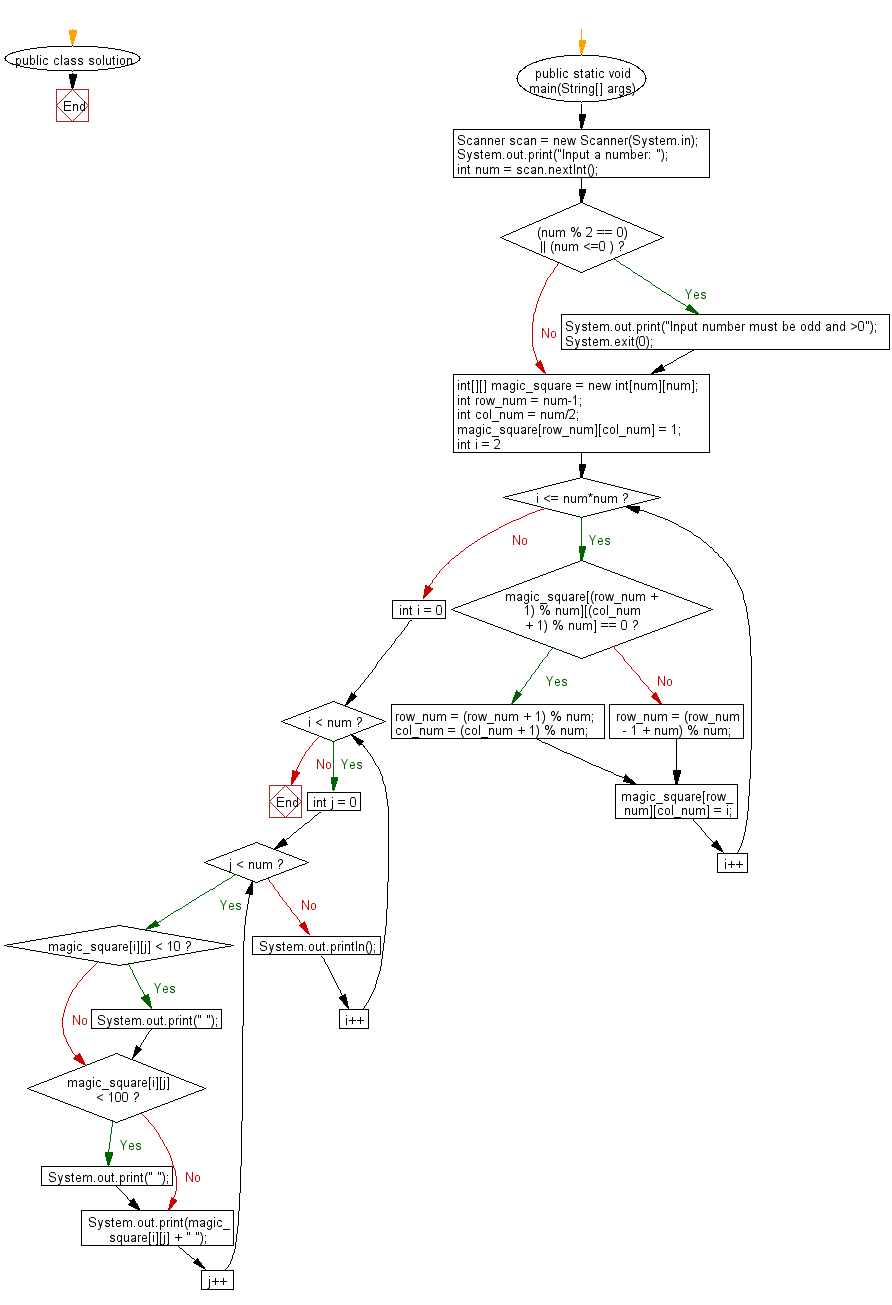﻿ Java Math Exercises: Generate a magic square of order n - w3resource# Java Math Exercises: Generate a magic square of order n

## Java Math Exercises: Exercise-19 with Solution

Write a Java program to generate a magic square of order n (all row, column, and diagonal sums are equal).
From Wikipedia,
In recreational mathematics and combinatorial design, a magic square is a n x n square grid (where n is the number of cells on each side) filled with distinct positive integers in the range 1, 2, ..., n2 such that each cell contains a different integer and the sum of the integers in each row, column and diagonal is equal. The sum is called the magic constant or magic sum of the magic square. A square grid with n cells on each side is said to have order n.

Sample Solution:

Java Code:

``````import java.util.*;
public class solution {

public static void main(String[] args) {

Scanner scan = new Scanner(System.in);
System.out.print("Input a number: ");
int num = scan.nextInt();
if ((num % 2 == 0) || (num <=0 ))
{
System.out.print("Input number must be odd and >0");
System.exit(0);
}

int[][] magic_square = new int[num][num];

int row_num = num-1;
int col_num = num/2;
magic_square[row_num][col_num] = 1;

for (int i = 2; i <= num*num; i++) {
if (magic_square[(row_num + 1) % num][(col_num + 1) % num] == 0) {
row_num = (row_num + 1) % num;
col_num = (col_num + 1) % num;
}
else {
row_num = (row_num - 1 + num) % num;
}
magic_square[row_num][col_num] = i;
}

// print the square
for (int i = 0; i < num; i++) {
for (int j = 0; j < num; j++) {
if (magic_square[i][j] < 10)  System.out.print(" ");
if (magic_square[i][j] < 100) System.out.print(" ");
System.out.print(magic_square[i][j] + " ");
}
System.out.println();
}

}
}
``````

Sample Output:

``` Input a number:  13
79  94 109 124 139 154 169   2  17  32  47  62  77
78  80  95 110 125 140 155 157   3  18  33  48  63
64  66  81  96 111 126 141 156 158   4  19  34  49
50  65  67  82  97 112 127 142 144 159   5  20  35
36  51  53  68  83  98 113 128 143 145 160   6  21
22  37  52  54  69  84  99 114 129 131 146 161   7
8  23  38  40  55  70  85 100 115 130 132 147 162
163   9  24  39  41  56  71  86 101 116 118 133 148
149 164  10  25  27  42  57  72  87 102 117 119 134
135 150 165  11  26  28  43  58  73  88 103 105 120
121 136 151 166  12  14  29  44  59  74  89 104 106
107 122 137 152 167  13  15  30  45  60  75  90  92
93 108 123 138 153 168   1  16  31  46  61  76  91
```

Flowchart:Java Code Editor:

What is the difficulty level of this exercise?

﻿

## Java: Tips of the Day

Parsing dates:

```import java.io.*;
import java.util.*;
import java.text.*;

String s = "2001/09/23 14:39";

SimpleDateFormat formatter = new SimpleDateFormat ("yyyy/MM/dd H:mm");
Date d = formatter.parse(s, new ParsePosition(0));
```